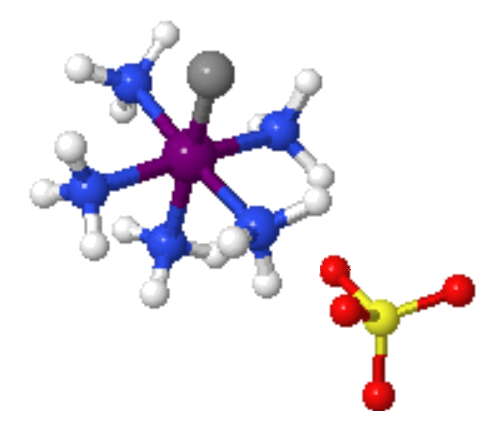Skip to main content

# Structural Isomers: Ionization Isomerism in Transition Metal Complexes

Coordination isomerism is a form of structural isomerism in which the composition of the complex ion varies. In a coordination isomer the total ratio of ligand to metal remains the same, but the ligands attached to a specific metal ion change. Ionization isomers can be thought of as occurring because of the formation of different ions in solution.

## Introduction

Ionization isomers are identical except for a ligand has exchanged places with an anion or neutral molecule that was originally outside the coordination complex. The central ion and the other ligands are identical. For example, an octahedral isomer will have five ligands that are identical, but the sixth will differ. The non-matching ligand in one compound will be outside of the coordination sphere of the other compound. Because the anion or molecule outside the coordination sphere is different, the chemical properties of these isomers is different.Figure $$\PageIndex{1}$$: Two Ionization isomers. The two isomers differ only which ligands are actually bound to the center metal. These two isomers are called pentaaquabromocobaltate(II) chloride and pentaaquachlorocobaltate(II) bromide.

The difference between the ionization isomers can be view within the context of the ions generated when each are dissolved in solution.

For example, when pentaaquabromocobaltate(II)chloride is dissolved in water, $$Cl^-$$ ions are generated:

$CoBr(H_2O)_5Cl {(s)} \rightarrow CoBr(H_2O)^+_{5} (aq) + Cl^+ (aq)$

whereas when pentaaquachlorocobaltate(II)bromide is dissolved, $$Br^-$$ ions are generated:

$CoCl(H_2O)_5Br {(s)} \rightarrow CoCl(H_2O)^+_{5} (aq) + Br^+ (aq).$

Note

If one dissolved $$[PtBr(NH_3)_3]NO_2$$ and $$[Pt(NO_2)(NH_3)_3]Br$$ into solution, then two different set of ions will be general.

• Dissolving $$[Pt(NO_2)(NH_3)_3]Br$$ in aqueous solution would have the following reaction

$[PtBr(NH_3)_3]NO_2 (s) \rightarrow [PtBr(NH_3)_3]^+ (aq) + NO_2^- (aq) \label{R1}$

• Dissolving of $$[Pt(NO_2)(NH_3)_3]Br$$ in aqueous solution would be

$[Pt(NO_2)(NH_3)_3]Br (s) \rightarrow [Pt(NO_2)(NH_3)_3]^+ (aq) + Br^- (aq) \label{R2}$

Notice that these two ionization isomers differ in that one ion is directly attached to the central metal, but the other is not.

Equations $$\ref{R1}$$ and $$\ref{R2}$$ are valid under the assumption that the platinum-ligand bonds of the complexes are stable (i.e., not labile). Otherwise, they may break and other ligands (e.g., water) may bind.

Example $$\PageIndex{1}$$

Are $$\ce{[Cr(NH3)5(OSO3)]Br}$$ and $$\ce{[Cr(NH3)5Br]SO4}$$ coordination isomers?

Solution

First, we need confirm that each compound has the same number of atoms of the respective elements (this requires viewing both cations and anions of each compound).

Element number of atoms in $$\ce{[Cr(NH3)5(OSO3)]Br}$$ number of atoms in $$\ce{[Cr(NH3)5Br]SO4}$$
$$\ce{Cr}$$ 1 1
$$\ce{N}$$ 5 5
$$\ce{H}$$ 15 15
$$\ce{O}$$ 4 4
$$\ce{S}$$ 1 1
$$\ce{Br}$$ 1 1

Now, let's look at what these two compounds look like (Figure $$\PageIndex{2}$$). The sulfate group is a ligand with a dative bond to the chromium atom and the bromine atom is a counter ion (\ce{[Cr(NH3)5(OSO3)]Br}\). For $$\ce{[Cr(NH3)5Br]SO4}$$, this is the the reverse.Figure $$\PageIndex{2}$$: $$\ce{[Cr(NH3)5(OSO3)]Br}$$ (left) and $$\ce{[Cr(NH3)5Br]SO4}$$ (right) are coordination isomers.

Yes, $$\ce{[Cr(NH3)5(OSO3)]Br}$$ and $$\ce{[Cr(NH3)5Br]SO4}$$ are coordination isomers.

Exercise $$\PageIndex{1}$$

Are [Co(NH3)5(SO4)]Br and [Co(NH3)5Br]SO4 ionization isomers?

Solution

In the first isomer, SO4 is attached to the Cobalt and is part of the complex ion (the cation), with Br as the anion. In the second isomer, Br is attached to the cobalt as part of the complex and SO4 is acting as the anion.

A hydrate isomer is a specific kind of ionization isomer where a water molecule is one of the molecules that exchanges places.

Solvate or Hydrate Isomerization: A Special kind of Ionization Isomer

A very similar type of isomerism results from replacement of a coordinated group by a solvent molecule (Solvate Isomerism). In the case of water, this is called Hydrate isomerism. The best known example of this occurs for chromium chloride "CrCl3.6H2O" which may contain 4, 5, or 6 coordinated water molecules.

• $$[CrCl_2(H_2O)_4]Cl \cdot 2H_2O$$: bright-green colored
• $$[CrCl(H_2O)_5]Cl_2 \cdot H_2O$$: grey-green colored
• $$[Cr(H_2O)_6]Cl_3$$: violet colored

These isomers have very different chemical properties and on reaction with $$AgNO_3$$ to test for $$Cl^-$$ ions, would find 1, 2, and 3 $$Cl^-$$ ions in solution respectively.

## Contributors and Attributions

• The Department of Chemistry, University of the West Indies)